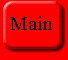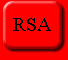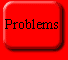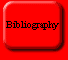## The History of Cryptography

This page is meant to give some insight into the history of cryptography, why it is needed, for what it is used, and what techniques have been used along with what measures have been used to break them.

Corporations around the world need to have some means of transmitting secret and classified data. Whether it is credit information or company strategies, there is a tremendous flow of data among many sources that needs to be somehow kept secret and cryptography is the way to achieve this. There have been many methods proposed and attempted. We start with a technique known as private key cryptography.

### Private Key Cryptography

Private key cryptography is perhaps the most traditional method of cryptography. One user would use a key that only s/he knew to encrypt a message using some function T. Perhaps the earliest cryptosystem was developed by the Greek historian Polybios. He used a grid of letters where each letter of the message was replaced by the two letters indicating the row and column in which the original letter lies. Here is a Polybios square with the English alphabet excluding the letter J.

 S Y D W Z R I P U L H C A X F T N O G E B K M Q V

Here P would be replaced by RD and G would be replaced by TW.      The next system we explore is the CAESAR system. Although Suetonius claims that Caesar used this system, Caesar actually replaced his Latin letters with Greek ones in a way that he did not make fully clear. The system that bears his name uses a simple technique and a private key. In this system, each letter is replaced by the kth letter beyond it, where k is the key. For example, say the message were the word "PRIVATE" and we use the private key k=4. Under the CAESAR system this would yield TVMZEXI. The user could then send this message to the recipient who also knew the algorithm and the key. This is a very simple example of a private key algorithm.

CAESAR is quite easy to break if an outsider were to know the algorithm, regardless of whether or not s/he had the key. A person would need only try a maximum of 26 permutations before finding an intelligible word. Most modern private key algorithms are actually quite more complex and thus difficult to break, but they all rely on the key remaining private. The problem then becomes the transportation of the key to the person who will be receiving the message. This is usually done by a courier, but the cost of frequently changing and distributing keys becomes quite expensive.

In 1977 the National Bureau of Standards created the Data Encryption Standard (DES) which was quite revolutionary at the time. DES was the first attempt at creating a universal encryption standard. DES was extremely successful and still remains as the most widely used cryptosystem of all time. Some of DES's main attributes are that the algorithm is extremely fast and it behaves according to the avalanche effect. What this means is that a small change in your key will yield very large differences in the produced cryptotext, thus making DES very difficult to break. Theoretical breaks of DES have been proposed, but they are still too costly and take to long to be considered true security risks. Machines designed specifically to break DES have been proposed, but their price estimates can range anywhere from millions of dollars to hundreds of millions of dollars and their performance estimates range from hours to a hundred days.

### Public Key Cryptography

This is where public key cryptography comes into play. The main idea behind public key cryptography is that not only can one make his/her algorithm public, one can make his/her key public as well. A person might publish their public key in a directory, and anyone who wishes to send that person secure information can encrypt that information using the public key and send it over insecure channels. Then when the cryptotext gets to the receiver s/he can decrypt it using their private key. This way, anyone can encrypt information, but only the person with the private key can decrypt it. Public key cryptography is very much like looking up someone's phone number in a large telephone directory. If you know the person's name, like having the private key, it is easy to find out their information. If, however, you only have their phone number you would have to search each entry one by one. Thus, the bigger the possible amount of names, the harder and harder it would become to find a person.

The idea of public key cryptography was first presented by Martin Hellman, Ralph Merkle, and Whitfield Diffie at Stanford University in 1976. They used a method which they referred to as the subset-sum problem, but which has been come to be known as the knapsack problem. The knapsack problem is based upon the NP complete problem that, given a container of some specific size and a set of distinct blocks, one can only find the set of blocks that fits exactly into the container using an exhaustive search or using special knowledge about the problem.

In the knapsack problem the public key given is an n-tuple A = (a1,a2,...,an) of distinct positive integers, as well as another positive integer k. The question is then which integers ai sum to equal k. For example, if we wanted to encode the message SECRET, we could convert each letter into binary format.

 S E C R E T 1010011 1000101 1000011 1010010 1000101 1010100

Since each letter corresponds to a binary number with 7 digits, we can set n = 7. To make the problem harder to break, we could combine 2 letters to make n = 14 and so on, but for this example we will keep n at 7. First we pick a private key made up of n numbers. For this example we will use A = (1,2,5,11,32,87,141). Notice that starting from the right, each number is bigger than all numbers to the left of it added together. This will become useful when decrypting the message.

Next we pick two more numbers: m such that it is greater than the sum of all ai and w which must have no common factors with m. We will use w = 901 and m = 1234. We use these to establish our public key by the equation ai = w * ai mod m, where ai is any single member in the public key and ai is the corresponding member in the private key. This gives us a public key of A = (901,568,803,39,450,645,1173).

Next we apply that public key to each letter. Encrypting just the letter S gives us:

B = 1 x (901) + 0 x (568) + 1 x (803) + 0 x (39) + 0 x (450) + 1 x (645) + 1 x (1173) = 3522

If we then send the number 3522 to the receiver, he can decrypt that using his private key and the equation B = B * w^(-1) mod m, where w^(-1) is the multiplicative inverse of w. This gives us: B = 3522 * (901)^(-1) mod 1234 = 3522 * 1171 mod 1234 = 234.

Now we are left to find the combination of A that will yield 234. This is easily done because of the property we mentioned before that each member of A is larger than all of the members to the right of it added together. In this example we get 141+87+5+1 or 1010011 which is the same as the S we originally encrypted. This solution can always be found without the key by trying all of the subsets of A, but if there are hundreds and hundreds of the numbers ai, then the problem quickly becomes unmanageable without the key.

### Why Does Public Key Cryptography Work?

Public key cryptography counts upon the assumption that there is not a "short-cut" to solving any of these algorithms. According to complexity theory, if one must try a tremendous amount of possibilities to find the right one, then the search will not be worthwhile or successful. All public key algorithms are thought not to be solvable in polynomial time. They are thought instead to be solvable only in exponential time. There is ongoing debate as to the question of whether there truly are problems that cannot be solved in polynomial time. This question is known as the P = NP question, where P is the set of all equations that can be solved in polynomial time and NP are the questions that cannot be solved in polynomial time. While most people currently believe that P does not equal NP, if that assumption were to be proven incorrect, it would mean that no public key algorithm is entirely safe. Even if P does not to equal NP, certain algorithms might have a polynomial time solution, or certain algorithms that are NP complete might have a backdoor, as was the case with the Hellman, Merkle, Diffie Knapsack algorithm.

While the knapsack problem remains NP complete, Adi Shamir of MIT found a way for a person without the private key to avoid doing an exhaustive search of the combinations. Shamir's attack finds the private key that will decrypt something encrypted with a certain public key, so one can find the key even before a message has been sent. Shamir's method finds a w and a m (numbers used to make the public key from the private key - see above) that will work as private keys. They may not be the same as the original, but they will work the same. The fact that the private key holder originally used a w and a m guarantee that at least one of each will exist, and the fact that they need not be prime suggests that there may be multiple pairs of w and m that will yield the same result. He finds w& m by graphing aiw(mod m) for all i = 1,...,n. It looks like thisIf we then superimpose several graphs for different i's and we look at accumulation points of minima of all of the curves, we can get w and thus calculate m.